#### A random sequence is probably dense

Suppose that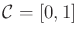. One of the simplest ways conceptually to obtain a dense sequence is to pick points at random. Suppose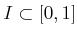is an interval of length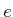. Ifsamples are chosen independently at random,5.3 the probability that none of them falls into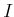is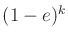. Asapproaches infinity, this probability converges to zero. This means that the probability that any nonzero-length interval in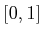contains no points converges to zero. One small technicality exists. The infinite sequence of independently, randomly chosen points is only dense with probability one, which is not the same as being guaranteed. This is one of the strange outcomes of dealing with uncountably infinite sets in probability theory. For example, if a number betweenis chosen at random, the probably that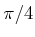is chosen is zero; however, it is still possible. (The probability is just the Lebesgue measure, which is zero for a set of measure zero.) For motion planning purposes, this technicality has no practical implications; however, ifis not very large, then it might be frustrating to obtain only probabilistic assurances, as opposed to absolute guarantees of coverage. The next sequence is guaranteed to be dense because it is deterministic.

Steven M LaValle 2020-08-14